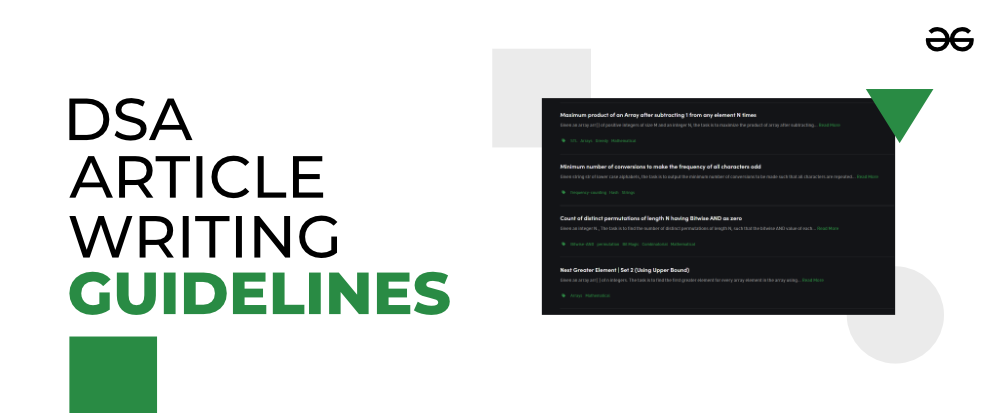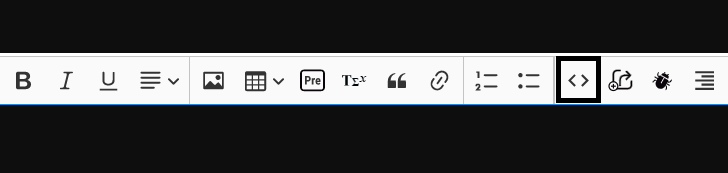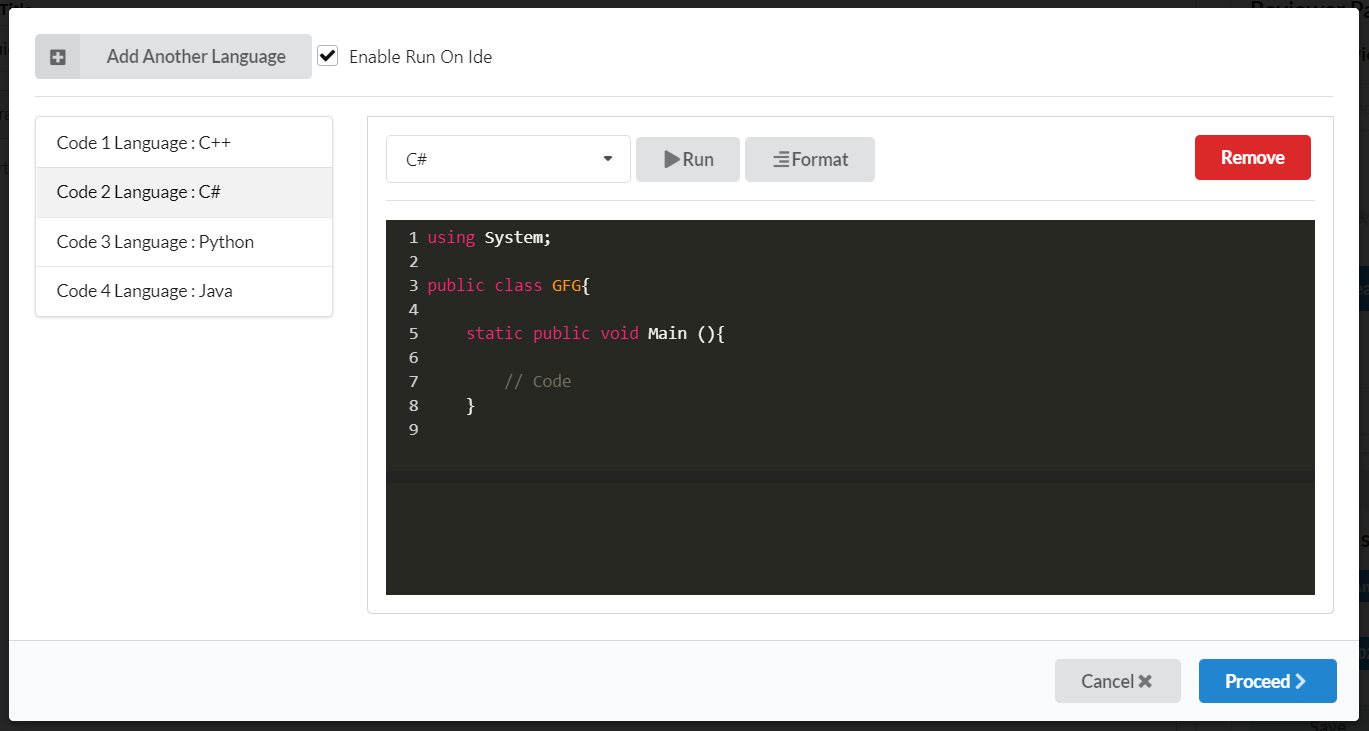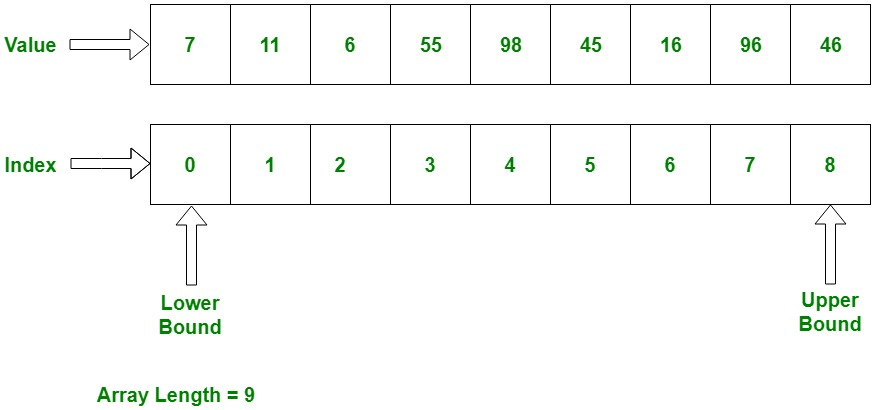GFG App
Open AppBrowser
Continue

# How to Write DSA Articles on GeeksforGeeks?

GeeksforGeeks provides all the coding enthusiasts an opportunity to showcase their programming and Data Structures & Algorithms skills by writing coding or DSA-based articles. However, a lot of individuals (especially college students or beginners) find it difficult to articulate their learnings & skills and contribute at GeeksforGeeks. But now the problem has got solved as this article will guide you through the entire process and guidelines of writing Programming/DSA articles at GeeksforGeeks.Let’s get started:

First and foremost, you need to know how to get started with article writing at GFG along with various other fundamental aspects like why should you contribute, where to write, etc. You can check out this link to know all these details in a comprehensive manner.

## How to verify if we can write an article?

Now, you need to check whether you can write an article on a particular topic/problem or not. You can do the same by following the below-mentioned steps:

1. Search the title and problem statement in the Custom search on GeeksforGeeks. If existing, don’t write.
2. Then search the title, problem statement, and examples (together and/or individually) on Google to find related existing links from other websites. If the same content is available somewhere as well, such as Codility, Codeforces, etc, this will lead to plagiarism and hence cannot be accepted.
3. Search some basic variations or generalized problem statements. If found, it will be considered plagiarism.

If the article passes the above points, the article can be written.

## Coding Article Format and Guidelines

Moving further, let’s check out the format and guidelines that you need to follow while writing a coding article at GeeksforGeeks. The programming articles should contain the following points:

1. Problem Statement
2. Examples (Two). Explanation of examples to get a proper understanding of how we achieved the result.
3. Links of prerequisites for the approach if there are any.
4. The approach used to solve the problem.
1. First, a basic idea or intuition
2. Then the step by step points regarding how this approach will work
3. Highlight any important observation or point using bold, blockquote, or pre.
5. Implementation / Code. If there are codes in multiple languages, the order should be C++, Java, Python3, C#. Please note that Python 2 codes are not accepted but Python 3 codes are accepted.
6. Time Complexity and Auxiliary Space, with the explanation of the terms used to express these.

Please try to keep the language simple, specific, and free from pronouns like I, you, we, etc

## Approach Format

If the approach is:

We create a mark[] array of Boolean type. We iterate through all the characters of our string and whenever we see a character we mark it. Lowercase and Uppercase are considered the same. So ‘A’ and ‘a’ are marked in index 0 and similarly ‘Z’ and ‘z’ are marked in index 25.

The above can be written as:

This approach is based on Hashing.

1. A Hashing data structure of boolean type is created of size 26, such that index 0 represents the character ‘a’, 1 represents the character ‘b’, and so on.
2. Traverse the string character by character and mark the particular character as present in the Hash.
3. After complete traversal and marking of the string, traverse the Hash and see if all characters are present, i.e. every index has true. If all are marked, then return true, else False.

## Sample Approach:

Problem – Print all unique combinations of setting N pieces on an NxN board

Approach: This problem can be solved by using recursion to generate all possible solutions. Now, follow the steps below to solve this problem:

1. Create a function named allCombinations, which will generate all possible solutions.
2. It will take an integer piecesPlaced denoting the number of total pieces placed, integer N denoting the number of pieces needed to be placed, two integers row and col denoting the row and column where the current piece is going to be placed and a string ans for storing the matrix where pieces are placed, as arguments.
3. Now, the initial call to allCombinations will pass 0 as piecesPlaced, N, 0 and 0 as row and col and an empty string as ans.
4. In each call, check for the base case, that is:
• If row becomes N and all pieces are placed, i.e. piecesPlaced=N. Then print the ans and return. Else if piecesPlaced is not N, then just return from this call.
5. Now make two calls:
• One to add a ‘*’ at the current position, and one to leave that position and add ‘-‘.
6. After this, the recursive calls will print all the possible solutions.

## How to Add Code in the Article?

Moving further, now you need to understand the process of adding the code in the article. It is as follows:To add code in multiple programming languages you can choose Add Another Language option as shown below. After that click on Proceed Button.In case your code does not compile or run due to any reason like libraries not supported by the compiler, you wish to demonstrate errors, etc, please uncheck Enable Run on Ide option to remove run buttons from the code. This button also avoids errors when your try to append output using the “Append Output” button which is just next to the ‘Add Code‘ button.

## Coding Standards

You need to ensure that you’re following the below-mentioned coding standards:

1) Function and variable names follow the camel case. For example, getMin(), getMax() and removeDuplicates(), isPresent, etc.

2) Driver code (or main function) does not contain any logic. It contains only input/output and function call.

3) Indentation should be done using 4 spaces.

4) Maximum 60 characters in a line so that the program is readable on mobile devices without much horizontal scrolling.

## C

 `// Below style should be avoided ` `int` `fun(``int` `a, ``int` `sumSoFar, ``int` `currSum, ``char` `val, ``int` `*result) ` `  `  `// The above should be written as ` `int` `fun(``int` `a, ``int` `sumSoFar, ``int` `currSum, ``char` `val, ` `        ``int` `*result)`

## C++

 `// Below style should be avoided ` ` ``cout << ``"Sample code to understand coding style for more readability of millions of readers"` `<< val; ` `  `  `// The above should be written as ` `cout << ``"Sample code to understand coding style for"` `     ``<< ``" more readability of millions of readers"` `     ``<< val;`

5) In case the code is written in multiple languages like Python, Java, and C/C++, the output of all codes should be the same.

6) Avoid the use of scanf (or cin) statements.

7) Spaces in while, if, else, for

## C

 `// There should be one space after while, no other ` `// spaces ` `while` `(i < 0)    ` `  `  `if` `(x < y) ` `{ ` `  `  `}`

8) There should not be any spaces for a function call or function declaration

## C

 `// No spaces after "reverse" or after "(" ` `void` `reverse(``char``* str, ``int` `low, ``int` `high) ` `{ ` `    ``while` `(low < high) ` `    ``{ ` `        ``swap(&str[low], &str[high]); ` `        ``++low; ` `        ``--high; ` `    ``} ` `}    ` `  `  `// Driver program to test above function ` `int` `main() ` `{ ` `   ``char` `str[] =  ``"geeksforgeeks"``; ` `   ``reverse(str); ` `   ``return` `0; ` `}`

9) Avoid the use of typdef.

10) Function names should be of the form “maxOfTwo()”, variable names should be of the form “max_of_two” or same as function name style. Class/Struct names should be of the form “ComplexNumber” or “SuffixTreeNode”. Macro names should be in capital letters like MAX_SIZE.

11) Avoid the use of static and global variables.

12) When we use cout, we must use a space between cout and “<<” and space between two “<<“. For example:

## C++

 `cout << ``"Sample"` `<< ``"Example"`

13) There should be space after comma in the declaration list and parameter passing.

## C

 `int` `x, y, z; ` ` `  `fun(x, y, z);`

14) There should be spaces in assignment operators

## C

 `// Should be avoided ` `int` `x, y=0; ` `  `  `// Should be followed ` `int` `x, y = 0; ` `  `  `// Should be avoided ` `x+=10; ` `  `  `// Should be followed ` `x += 10;`

15) At the beginning of every program, please write a line to tell the purpose of the program:

## Java

 `// Java program to illustrate sum of two numbers`

Note: It is strongly recommended to add time complexity after your code in data structures/algorithm articles.

## Image Creation Guidelines

The image creation guidelines are as follows:

• The name of the image must be Relevant. For example, if an image depicts”: “Adding of two numbers using Linked List”, the title of the image must be: Adding of two numbers using Linked List.
• In case of images being built using the packet tracer, please send the screen recording in place of the image if possible.
• In case of screenshots, please add GeeksforGeeks (or GFG) somewhere in output. For example, it may be folder name, image title, etc.
• It is mandatory to create your own images using some image drawing tool (For ex: Google Drawings, MS Paint, https://www.draw.io/). Images MUST NOT be taken from any other source to avoid any copyright issue. You can follow the below guidelines to create images in GeeksforGeeks style:
• The boundary/outline of the image should be of black color.
• The text to be written should be of green color.
• Our priority should be to use a white background, if other background colors are needed, please use green as background and white color in the text.
• Red color should be used to show movements, representations, variations, and other things.

Please see below the example image, to get a clear idea about the image creation of an Array:### Important Points (With Examples)

1. If the approach is quite simple or concise then there is no need to mention step-by-step points for solving the problem – the steps to solve the problem can be covered in that single paragraph itself. For example – check out this article: Sort a linked list after converting elements to their square

2. Add Time Complexity and Auxiliary Space after your code in data structures/algorithm articles. Check out the article for reference purposes: Print all unique combinations of setting N pieces on an NxN board

3.  While using an Efficient Approach for solving a problem, you first need to tell why it is better than the Naive/Old Approach. For example – check out this article: Count of pairs (arr[i], arr[j]) such that arr[i] + j and arr[j] + i are equal

4. A few more sample articles:

Note: Problem directly taken from Codility & Codeforces cannot be published due to copyright issues.

My Personal Notes arrow_drop_up